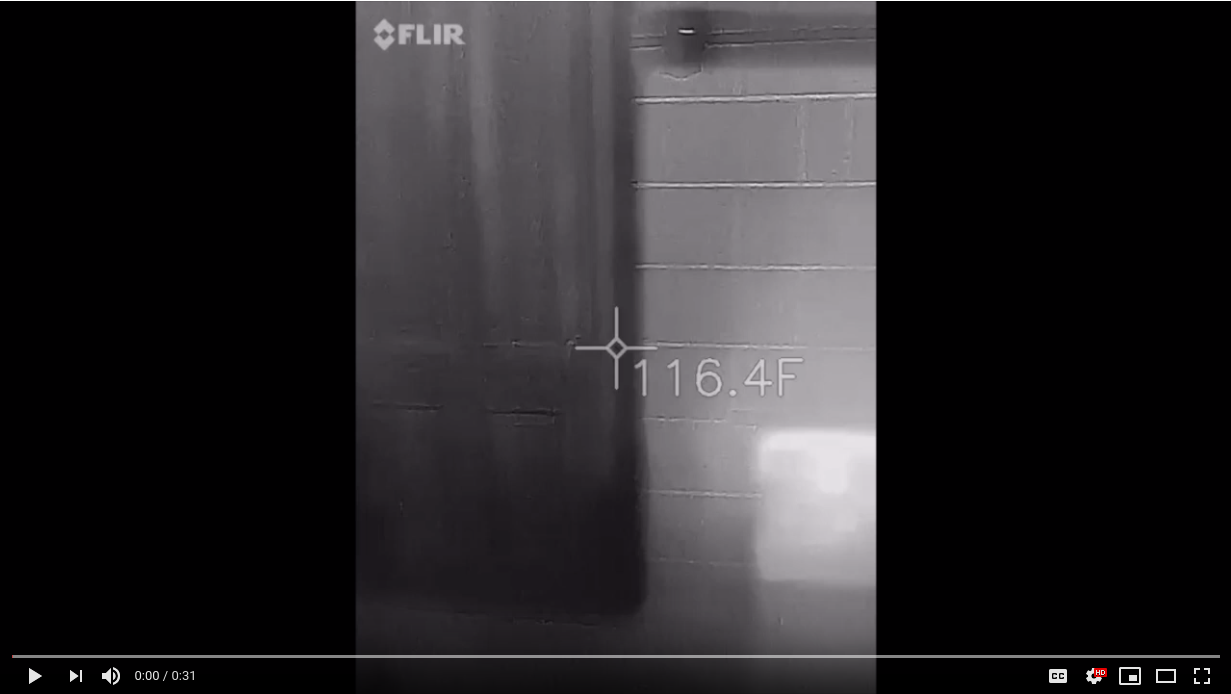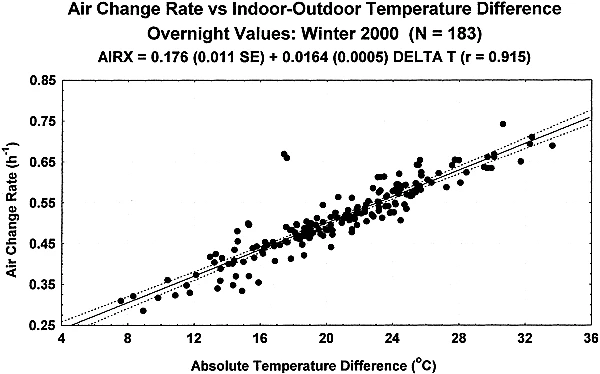My brother Mark bought a trailer sauna and was tinkering with various options on the sauna, wondering how various changes would affect the thermal dynamics of the sauna. I tried to respond with some first order calculations, but it was fairly clear they were not accurate enough. This inspired me to build a differential equations model of a sauna in Julia, which can be accessed on saunasim. Check out a thermal video of steam throwing in a sauna here:## Start up

The massive upfront cost of the project is research. Basic questions like ‘How hot is a fire?’ and ‘How much heat is it producing?’ must be run down. Picking a set of assumptions in order to build an interesting model that still won't take years to build is key. This means choosing boundary conditions and dividing the problem into lumped components.

### Components

Elements of the sauna include:

• Fire
• Stove
• Air temperature
• Air humidity
• Walls and furniture of the room
• Floor of the sauna (concrete)
• Air outside (both temperature and humidity)
• Mass of water on stove
• Temperature of water on stove Since Sauna Sim is a lumped sum model, each of these elements is considered to be in a uniform state. There are many other possible variables to consider, such as the effect of adding a water heater. Another bit of complexity to add would be a few floor and ground elements to simulate the heat transfer down into the ground.

### Boundary Conditions

One way to make the system simpler to deal with is to assume a constant value for some components of the system and to drive some components with functions. In the default scenario:

• Floor of sauna is assumed to be 50 °F
• Outside temperature is assumed to be 50 °F
• Outside relative humidity is 30%
• The fire grows to a maximum temperature of 1000°F and a radius of .3 meters

## Heat Exchange

### Heat transfer equations

Here is a short review of heat transfer. There are only a few equations that drive this process. For all equations below A is area, L is length, T is temperature in Kelvin.

#### Conduction

Conduction occurs when heat moves through physical contact with objects. In the sauna, the main conductive aspect is the walls conducting heat to the outside. Effective insulation will greatly reduce this mode of heat transfer.

$k\frac{(T_{hot}-T_{cold})A}{L}$

where $k$ is the conduction coefficient, which is fairly straightforward to measure for many materials. To learn all you probably need to know about thermal conduction, consult Wikipedia..

#### Convection

Convection is the mode of heat transfer due to fluid flows. This mode of heat transfer is extremely common in a sauna. The heat transfer from a burning hot steam to your shoulders is one. Heat transfer from hot gas rising off the fire to the stove is another.

$h(T_{hot}-T_{cold})A$

where $h$ is the convective coefficient. Convective coefficients can be approximated through a fairly complex process of determining Reynold's numbers and Nusselt's numbers. I used reference numbers to save time. To learn all you probably need to know about thermal convection, consult Wikipedia.

Radiation is the mode of heat transfer due to electromagnetic radiation. It’s heat moving like light, instantaneously, through the atmosphere. If you feel the heat coming off of something hot, it is probably radiation. The burning sensation in your shins from an oversized stove (cough, Benda's, cough) is from radiation.

$\sigma\epsilon A (T_{hot}^4 - T_{cold}^4)$

where $\sigma$ is the Stefan–Boltzmann constant (some universal constant), $\epsilon$ is the emissivity (black top has a high emissivity, white shirts have a lower one). I assumed that $\epsilon=1$ for the purposes of this model. Wikipedia.

Heat is also transferred when hot air leaves the room and cold air flows in. Since our sauna is not leak proof, a certain amount of the air in the room turns over with every time increment. This rate is associated with the difference in air temperature. Nature had a fairly solid article on the turnover rate for a house, and I adjusted it (upwards) for the surface area to volume ratio of the sauna under consideration. All smaller rooms have a larger surface area to volume ratio compared to larger rooms.#### Phase change

Throwing water on the stove causes heat to convect into the water on the stove rapidly turning it into steam. This phase change heats the air and stings your ears. Since this is also a mass transfer, it is also advection. The steam coming off the stove is assumed to be 212°F as there will be minimal heating of the steam after it boils.

## Stove - Fire Heat exchange

By my figuring, the two dominant ways heat transfers from a fire to a stove are convection and radiation. Conduction will play a small role, but I ignored it for the purposes of this model. Radiation exchanges at the difference of the fourth powers of temperature, while convection exchanges linearly with temperature. Convection:

$h(T_{hot}-T_{cold})A$

$\sigma\epsilon A (T_{hot}^4 - T_{cold}^4)$

One thing to note: the hotter your stove, the less heat transfer from the fire to the stove. That means you have to add more and more fuel as you attempt to reach higher temperatures in the sauna.Radiation heat exchange is dominated by the larger term, making initial changes in the lower temperature less important. Convective heat exchange is linear with the difference in temperature. This will cause radiation to become a more and more important component of the heat transfer as stove temperature climbs.

## Humans getting hot in the sauna

I have another full blog post on humans getting hot in the sauna. Read it here.

## Next steps

The next steps to take with the sauna would be to calibrate the model off of an existing sauna. A few thermocouples and a humidity monitor would provide some very interesting data, as well as periodic snapshots with the thermal camera.

## So what?

Saunas are complex dynamical systems, with relevant humidity, temperature, and air flow. Getting a handle of how all these systems work together takes quite a bit of research. However, the benefits of simulation for an industrial project can be immense. For example, the trade-off between more capital and greater efficiency can be explored with a model. Contact Laurium Labs if you need help getting a handle on your problem.